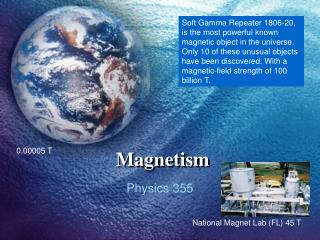DownloadDownload PresentationMagnetism

# Magnetism

Télécharger la présentation## Magnetism

- - - - - - - - - - - - - - - - - - - - - - - - - - - E N D - - - - - - - - - - - - - - - - - - - - - - - - - - -
##### Presentation Transcript

1. Soft Gamma Repeater 1806-20, is the most powerful known magnetic object in the universe. Only 10 of these unusual objects have been discovered. With a magnetic-field strength of 100 billion T. Magnetism 0.00005 T Physics 355 National Magnet Lab (FL) 45 T

2. From whence does magnetism come? 1. No answer from classical physics... 2. What is the role of conduction electrons? 3. What is the effect of localized electrons? 4. How do we make powerful permanent magnets?

3. Magnetism Electron- Electron Interactions Quantum Mechanics

4. Classically, the change in energy of a particle with respect to time is • ...But in a magnetic field, the force on the electron due to the field is • always perpendicular to the electron’s velocity. This would mean that • the energy of a system should not be able to change in the presence of • a magnetic field – Yet, it does!! Quantum Mechanics Why is magnetism a quantum effect?

5. Quantum Mechanics Orbital Angular Momentum All known magnetic properties originate from the attributes of charged particles. Magnetic dipole moment The right-hand rule determines the direction of the magnetic moment of a current-carrying loop. The direction of the electron's angular momentum vector L can be obtained using the right hand rule for angular momentum.

6. Zeeman Effect The Effect of Magnetisation on the Nature of Light Emitted by a Substance P. ZeemanNature, vol. 5511 February 1897, pg. 347

7. Zeeman Effect Pieter Zeeman 1865-1943 When considering atomic spectra, the atomic energy levels, the transitions between these levels, and the associated spectral lines are produced with no magnetic fields influencing the atom. If there are magnetic fields present, the energy levels are split into a larger number of levels and the spectral lines are also split. This splitting is called the Zeeman Effect.

8. The total Hamiltonian of an atom in a magnetic field is: • where H0 is the unperturbed Hamiltonian of the atom, and the sums over α are sums over the electrons in the atom. The term • is the spin-orbit interaction for each electron (indexed by α) in the atom. If there is only one electron, the sum contains just a single term. The magnetic potential energy • is the energy due to the magnetic moment μ of the α-th electron. It can be written as a sum of the contributions of the orbital angular momentum L and the spin angular momentum S, with each multiplied by the appropriate Landé g-factor, gL or gS. By projecting the vector quantities onto the z-axis, the Hamiltonian may be written as • where the approximation results from taking the g-factors to be gL = 1 and gS 2. The summation over the electrons was omitted for readability. Here, Jz = Lz + Sz is the total angular momentum, and the spin-orbit (LS) coupling term has been combined with H0 and written as Hat.

9. Zeeman Effect  MJ = +½ 2 1 MJ = ½ The probability that an ion is in a state with Jz = MJ is proportional to

10. Quantum Mechanics Spin Quantization Otto Stern 1888-1969 Walther Gerlach 1889-1979

11. B Magnetism Magnetic Susceptibility H – magnetic field intensity (external) M – magnetization, dipole moment per unit volume (internal)

12. Magnetism Magnetic Classifications • Paramagnetic • Diamagnetic • Ferromagnetic

13. Example • Suppose a long cylindrical specimen with a uniform magnetization M parallel to its axis is placed in a uniform applied magnetic field BA, applied along the axis of the cylinder. Suppose the specimen has a magnetic susceptibility . • Find the magnitude of the magnetization M, the magnetic induction B and the magnetic field intensity H. M

14. Example - Answers • If the material is paramagnetic, then the magnetization is in the same direction as the applied field and it produces a field 0M in the same direction as the applied field.

15. Example - Answers • If the material is diamagnetic, then the susceptibility is negative. The results are the same as before, except that B < BA.